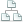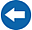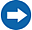Home >Tips >Excel 2016 from Scratch >Percentage, sums of sumsSite map

# Excel 2016 from Scratch Exercise Percentage and sums of sums

## An Excel tutorial by Peter KalmstromIn this demo in the Excel 2016 from Scratch series Peter Kalmstrom explains how to create a formula that calculates percentage. He also shows how to calculate sums and sums of sums.

Peter uses a conveyour bid as an example in this exercise, and when he has caclulated all sums he creates a formula that calculates how much the labor costs in percent of the total.

### Exercise

If you want to try the exercise yourself, please download the Excel file Peter uses in the demo!

### Content

This is what Peter shows in the demo below:

• How to create autosum formulas and select the cells to include.
• How to calculate a sum of the sums.
• How to create a division formula and show the result in percentage by formatting the cell as percentage..
• How to add a percentage sign to a number.
Peter uses Excel 2016 for his demo, but the Excel basics are the same for earlier versions of Excel.